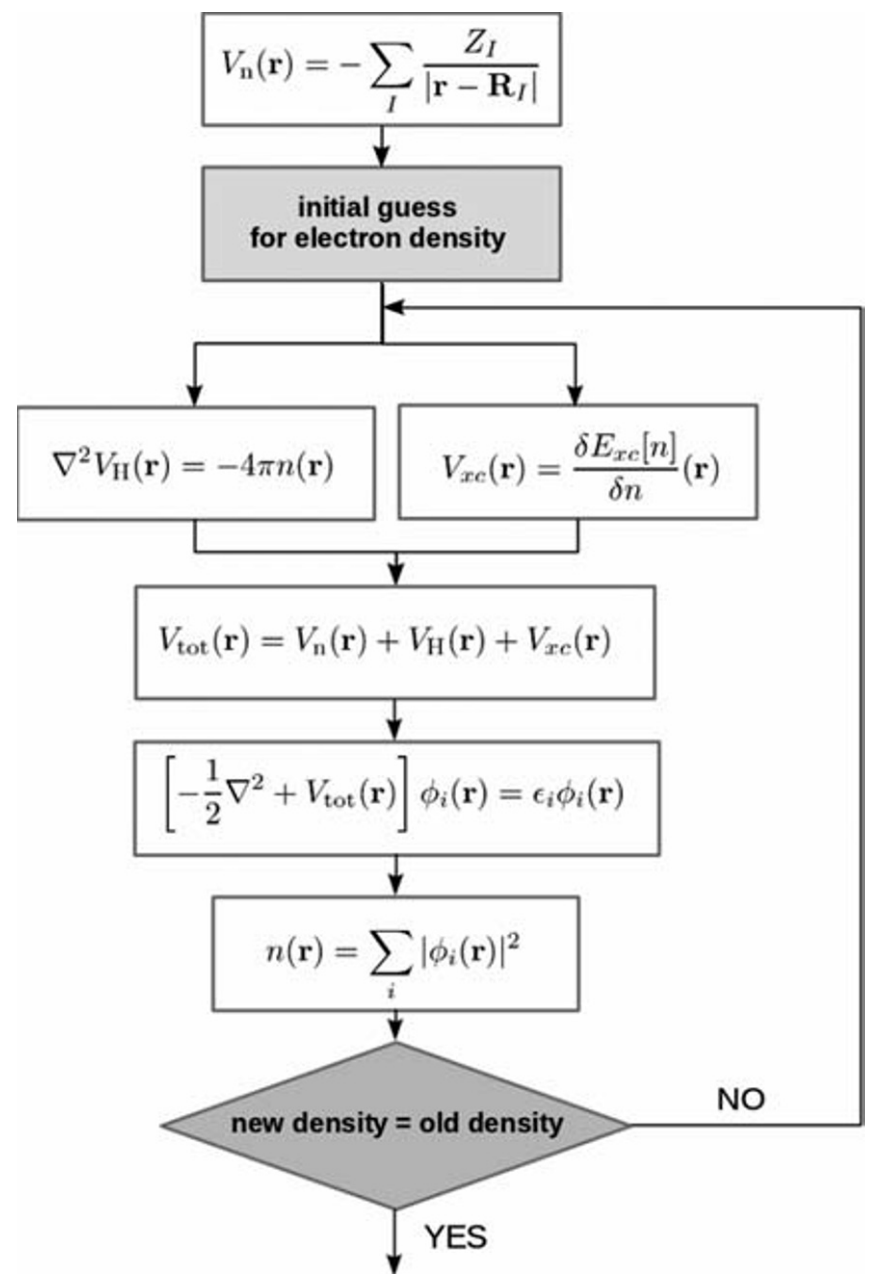# 1) Basics of Density Functional Theory, DFT

Here, I am trying to summarize what I have learned about materials modeling using the DFT method.

My focus is on Quantum Espresso software. To do that I have started to learn the basics of DFT. My sources are:

 Giustino, F. (2014). Materials modelling using density functional theory: Properties and predictions.
 Quantum Espresso tutorials section :
https://www.quantum-espresso.org/resources/tutorials
 From Atoms to Materials: Predictive Theory and Simulations (online course on edx.org, from Purdue University)
https://courses.edx.org/courses/course-v1:PurdueX+MSE550x+3T2017/course/

# 1. First Principles or ab intio model

Simply refers to modeling of materials without using the experimental data or without fitting the data coming from experiments. If we know the fundamental characteristics and theory of materials and elements and put them on the paper with mathematical equations, we will get numbers or other equations that in the real world are a resemblance of experimental values.

The fundamental theory of materials, elements, or chemicals is Quantum Mechanics. Quantum mechanics is giving you numbers and equations that you can use to put the reality on the paper and show it by mathematical expressions.

The problem is the complexity of the equations and most of the time the need for supercomputers to solve them. These equations come from the complicated Schrödinger equation. When the words “Supercomputers and Calculations” appear, we should understand that the materials modeling is an interdisciplinary field that consists of materials science, physics, chemistry, mathematics, numerics, and software engineering.

So what? Why do we want to model the real world? Let it be as it is. right? The answer is the prediction. There are many scientists who want to predict their experimental results or predict their materials properties beforehand. That is why materials modeling becomes important. Some times you don’t have access to a specific chemical, or synthesis parameters are very difficult to control, or you are not sure about the final product’s properties.

# 2. Density Functional Theory (DFT)

DFT is a method of calculations to approximately solve the Schrödinger equation. It was established based on the assumption of electrons in the materials to be like an inhomogeneous gas. DFT is giving the ability to the current technologies to model the materials. DFT is still being optimized in terms of accuracy and calculation time.

As an example of a modeling application in designing new materials, we could mention the catalyst design for hydrogen evolution reaction, HER. The key point in this example is that the Gibbs energy for hydrogen adsorption on the catalyst should be around zero to be a good candidate to replace expensive platinum catalysts. DeltaG of H adsorption can be calculated by DFT by calculating the total energy of the system before and after chemically adsorption of H. Also, the stability of the imaginary catalyst can be calculated by the related Gibbs energy change as a result of degradation.

3. Schrödinger equation

3.1.Particles Interactions
There are electrons and nuclei, that’s it. We should find their interactions and then find the whole energy of the package. Electrons repel each other, nuclei repel each other but electron and nuclei attract each other. These repulsions and attractions (Coulomb Interactions) are sources of internal energy. If we take “d” as the distance btw the particles, the energies are :eq 1

3.2.Many body Schrödinger equation
Describing quantum particle behavior needs determining the wavefunction of that particle in any area or point that we are interested in ( we are here to find the behavior of particles: any kind of behavior like electronic structure). In other words, the particles have different behaviors at different points, r,  of the space, to find that behavior we need to determine the wave function at that point.

3.3.Wave Function(we will tell it \psi )

it is the function of position. It also demonstrates the probability of finding the electron at position (r). if the wave function is zero at a position, the electron is not there.How do we determine the wave function? by solving the Schrödinger equation. If we imagine that we are studying the stationary electron-ic properties of materials, we can use the time-independent Schrödinger equation.eq 2
E is the energy eigenvalue of that stationary state.

There are some important definitions that are needed here:is the probability of finding the corresponding particle at the position r.

3.4.Schrödinger equation for a single electron

If we simply consider that an electron is in a box with the potential of V(r) around it we can write: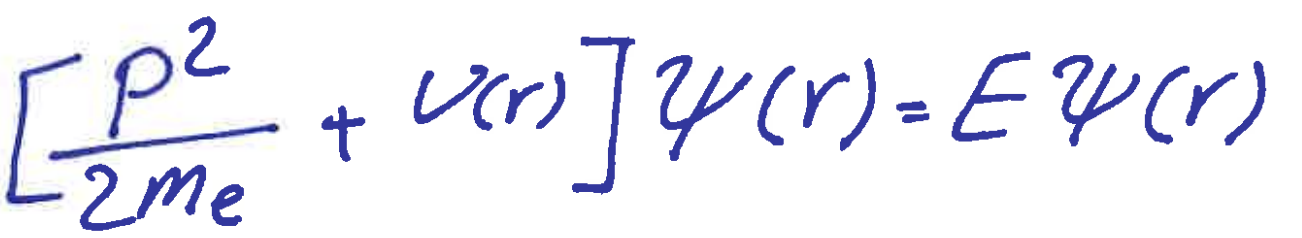eq 3
m_e is the electron mass and p is the momentum.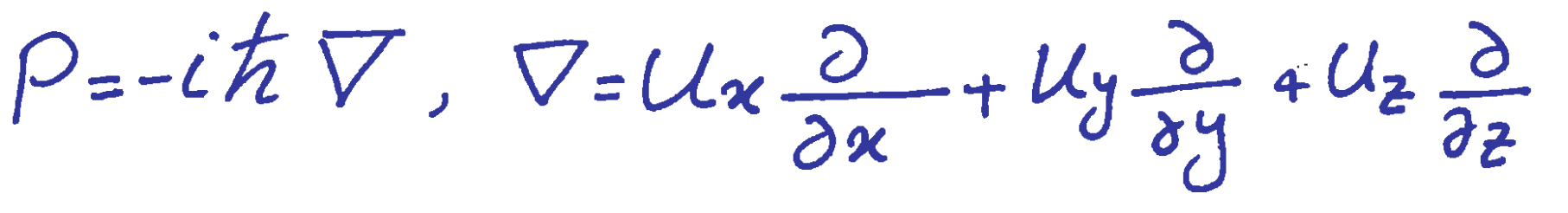h is the reduced Planck constant.

If we calculate the equation 3 and find the \psi_0 as our answer for the lowest energy of a single electron system then the electron distribution can be given by:3.5.Schrödinger equation for many electrons and nuclei

However, for systems with many nuclei and electrons, it is not accurate to just linearly add singular contribution from particles. Here we need many-body wave function \PSI.If r is the position of electrons and R is the positions of nuclei, we can write: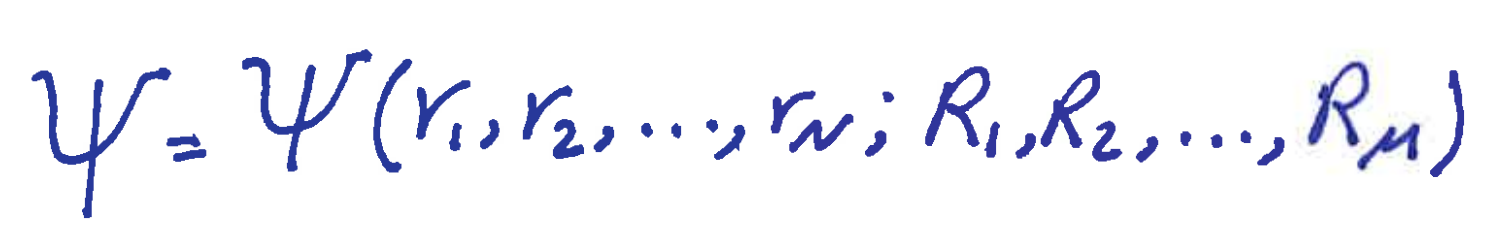is the probability of finding the corresponding particle at the position r. If we want the probability of, at the same time, finding the 1st electron at r1 and 2nd electron at r2 and so forth we can write:But most of the time we want to find the total charge distribution probability. It means the summation of all the above probabilities for all arrangements. Here we sum these:

1. Probability of finding the electron 1 at all r, while 2 is at r2, 3 is in r3, and…
2. Probability of finding the electron 2 at all r, while 1 is at r1, 3 is in r3, and…
3. Probability of finding the electron 3 at all r, while 1 is at r1, 2 is at r2, and…

The first one is:eq 4

3.6.Electron or charge density in the material

Electron density at the position r (finding any electron at r) is equal toAs electrons are not such particles that we can label them and distinguish them from each other, all the P s are similar and we can write n = N*p.
N = electron numbers, so
n =Integration n (charge density) all over the materials gives the number of electrons.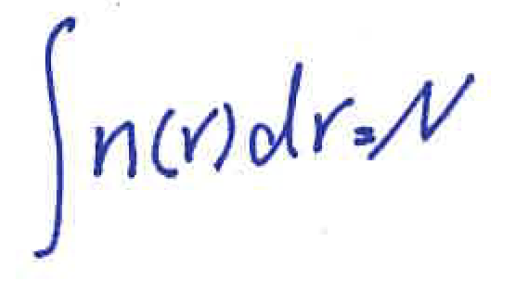As summary:

Charge density can be defined as how dense is the charge distribution in the material. The denser the charge distribution, the more probable that we can find the electron in the position r, the higher the value of n.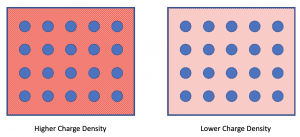The summation (integration) of the charge density throughout the materials gives the number of electrons. Note: the integration of wavefunction throughout the material is normalized to one.

3.7.Finally, the equation

Now we can write the many-body equation.kinetic energy is the total kinetic energy of all electrons and nuclei. Potential energy is the total attraction and repulsion energies between electrons and nuclei.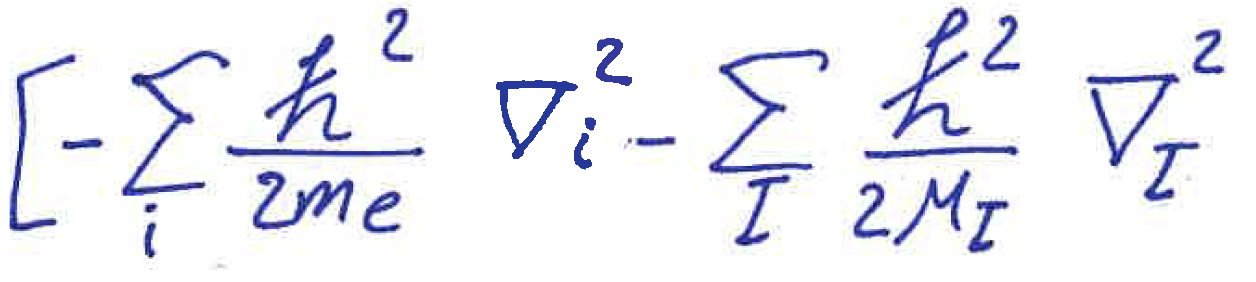the kinetic energy of both types of particlespotential btw electrons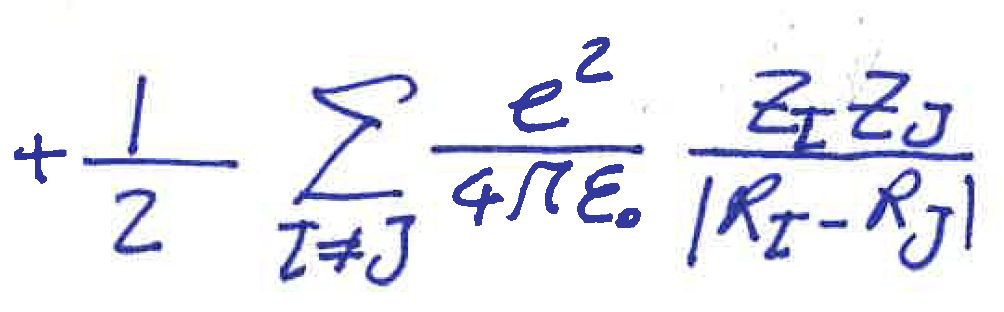potential btw protons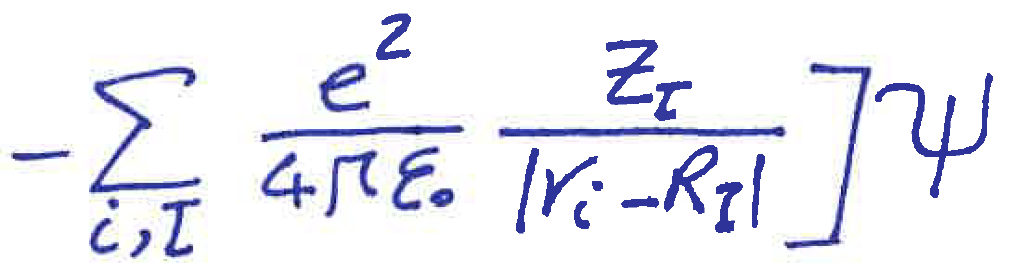potential btw electron and protonsThis equation is everything we need. The stable materials without changing by time without the external field.

By solving this equation, the lowest energy, E,  of the system could be driven. Then from this energy, we could find all the properties of the material like elastic behavior, enthalpy of formation so forth. BUT, most of the time it is impossible to solve it. :((((( WHYYYY????

3.8.Simplifying the equation appearance

If we divide all parts of the above equation by the Hartree energy the shape of the equation would be simpler. (Hartree energy is the lowest potential energy between electron and proton in the Hydrogen atom).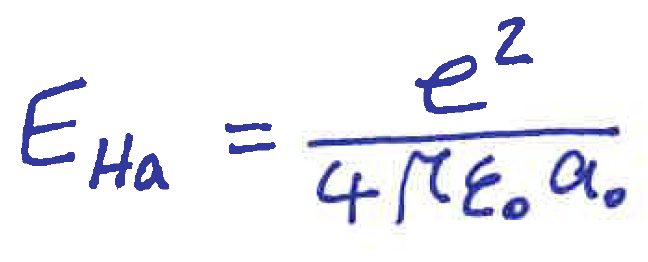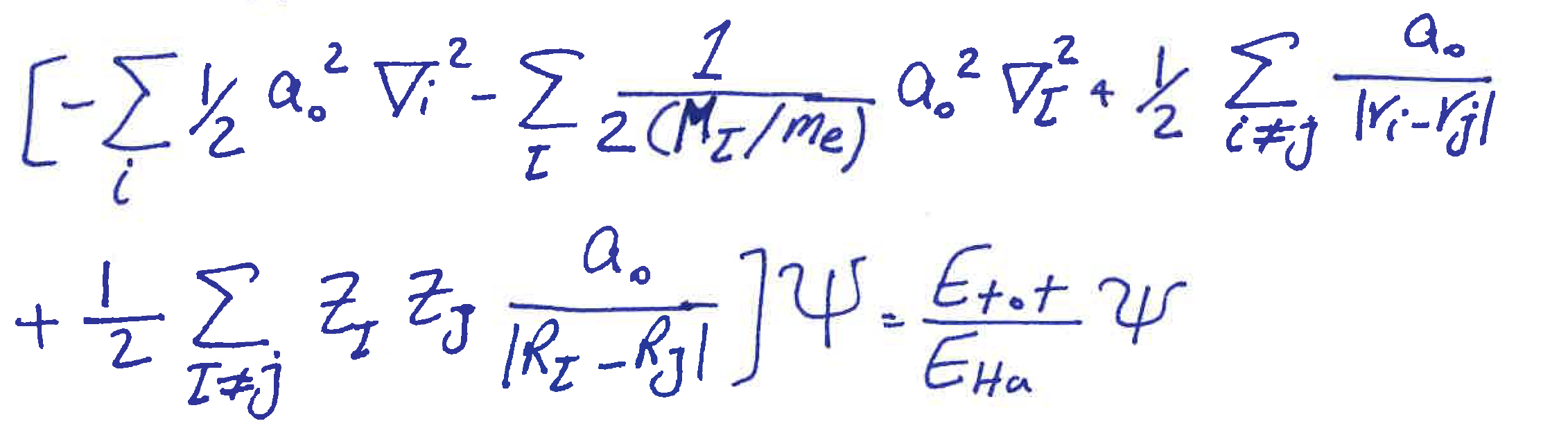If we take the Hartree energy as 1 unit, the a_0 as 1 bohr, and m_e as 1 a.u., the equation becomes simpler: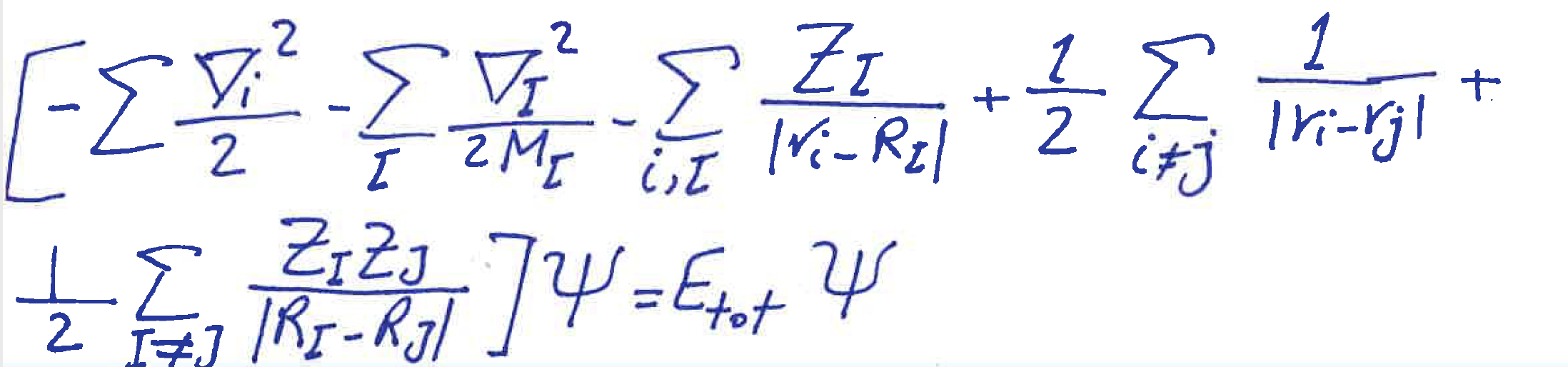eq 5
Here, there are just the atomic Mass and atomic Numbers are needed.

3.9.Approximation:

• Nuclei are not moving in solids and molecules so we can ignore the kinetic energy of nuclei, and add the potential energy btw nuclei as a constant number to the right-hand side of the equation.
• Also, the 2nd term from the left is the potential that the proton imposes on the electrons. We will name this as V(r).eq.6H is the Hamiltonian.

So far we just defined the proper equation that we can use to model the materials. However, the story begins here: How should we solve this equation? How should we deal with the interactions btw the electrons (the 3rd element from the left)?

It is useful to point out some concepts and definitions:

1. Pauli exclusion principle says that when we exchange the spin or position of two electrons, the sign of their wave function is changed.
2. interaction of the electrons have a strong effect, so we can not ignore it.
3. the clouds of electrons in the materials, themselves create a potential and this potential affects electrons. An electron in this field of potential has “Hartree Potential.(V_H)

4.1.Simplifying the equation:

• We can imagine the electrons are not interacting with each other first, which means removing the third element, and just put the Hartree potential on them. In this situation, the equation for single-electron becomes: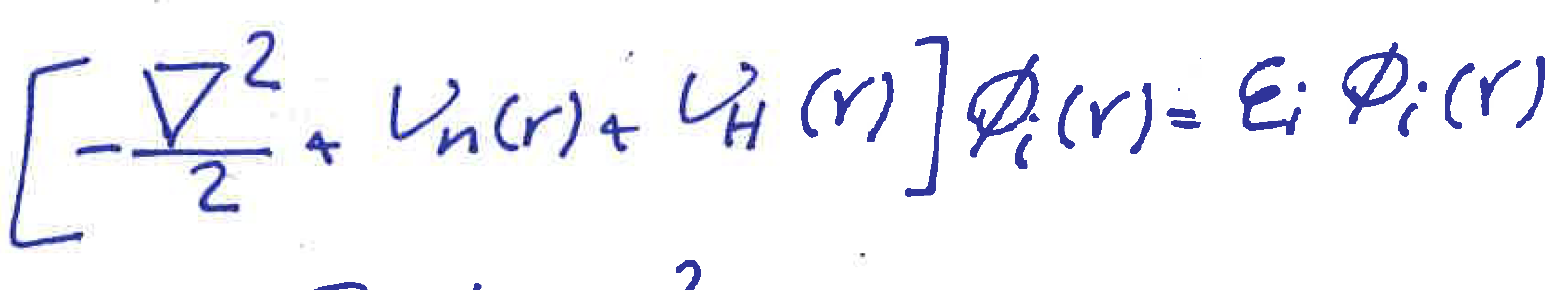eq.7
• Solving this equation gives the wave function (\phi_i) of one electron and we can write the density of charge as: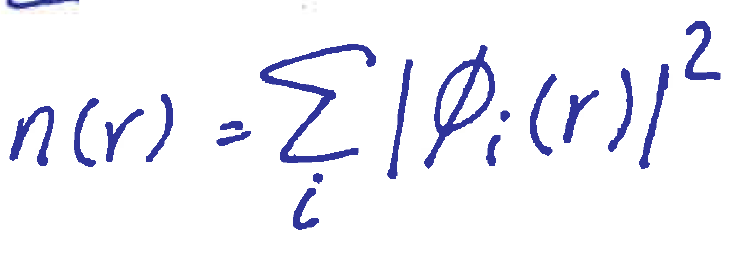eq.8
• The hartree potential satisfy also this equation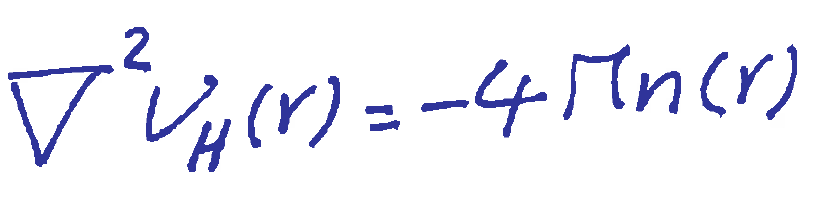• This approach is called mean-field approximation. In which, the electrons are not interacting with each other and are considered to be as classical particles.

4.2. Step by step adding the other potentials to the equation

• We said that eq.7 comes from classical mechanics. To come to the quantum territory, let’s add, step by step, the potentials that matter here.
• One of the potentials that should be added is the one that does not let two electrons occupy the same state (Pauli exclusion principle). We call it V_x.
• There is another potential that comes from the repulsion btw the electrons. This potential decreases the probability of finding an electron near one other electron. It means the lower distance the lower probability of finding. We call this potential the correlation potential and show by V_c.
• The nature of these two potentials is still unknown, but they exist.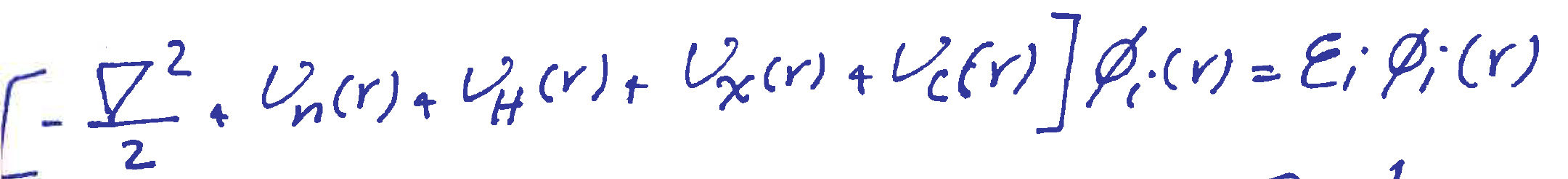eq.9
• This is the equation for single electrons who are in a solid. by solving this we can find the whole electron density by eq.8.

Until this point, we changed the many-body equation to the summation of single-electron equations with expressing the potentials that are imposed on single electrons in a solid. The next part, (DFT), is finding these potentials in ground states.

5. Density Functional Theory (DFT)

• Before using the previous equations, let me say that the main part of the DFT is based on this claim (by Hohenberg and Kohn):
• The ground state energy (the lowest level of energy) of materials is the functional of the electron density
E = F[n]
• other levels of energy (excited states) are the functionals of the many-body wave function of that state.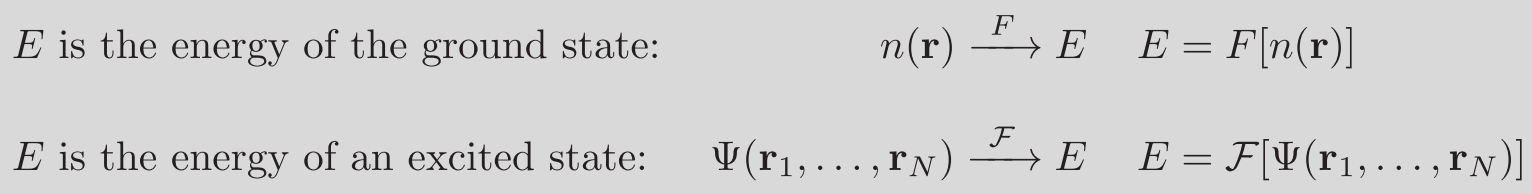• We had that the hamiltonian can be written: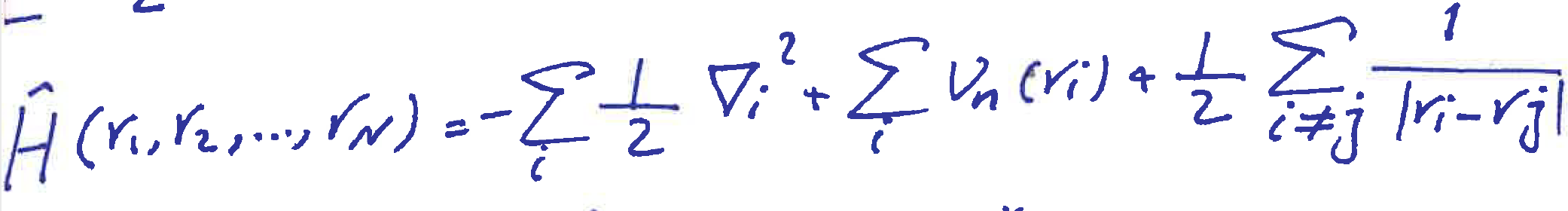• The Energy is written in this form (an experimental :• So: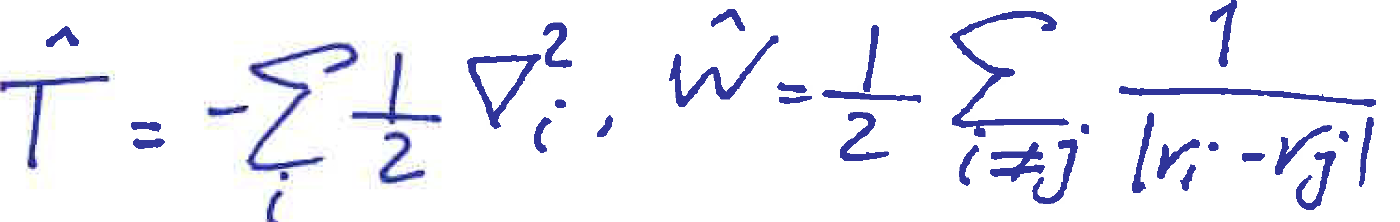>>>>>>>>>>>>>>>>eq.10
• The first term of the energy is the energy that comes from the nuclei, and as it is shown, it depends on the electron density.
• The 2nd term (kinetic and coulomb energy) dependence on electron density is not clear that much.
• We can again change the 2nd term of the energy equation here into the summation of the energies of the independent electron (kinetic and coulomb) plus unknown parts.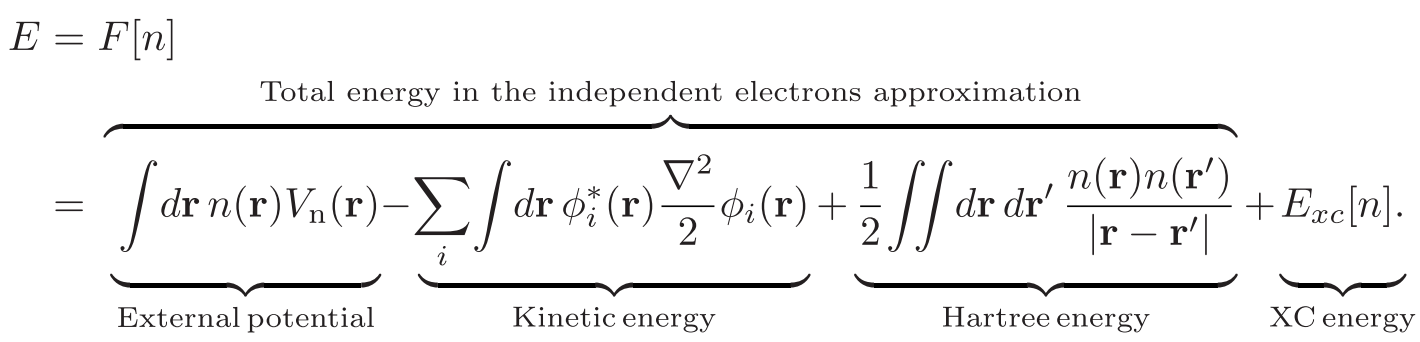• E_xc is the unknown part of the energy that is also the functional of electron density and as I said it should be approximated:

At the ground state, the electron density (the ground-state electron density) minimizes the energy (was obvious as we said: at ground state the energy is the functional of the electron density). We call this derivative equation as variational principle: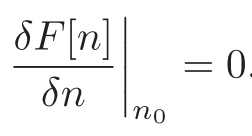Mathematical equations and other stuff lead to: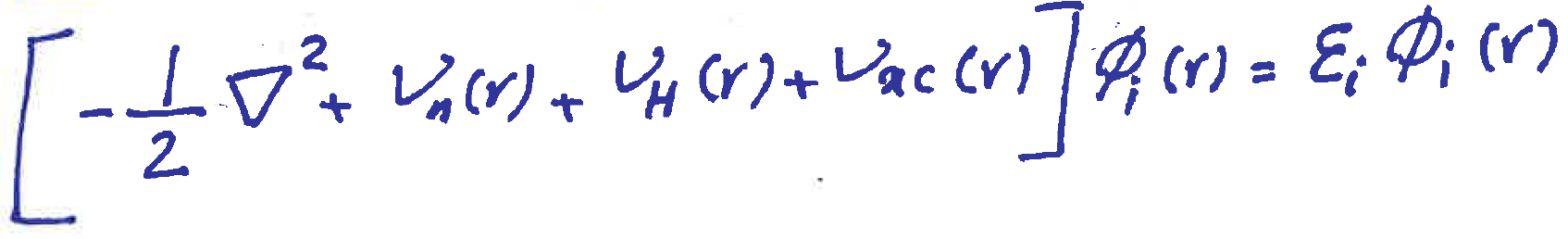this is the Kohn-Sham equation and is similar to the equation written for the single electron in the many-body environment. The point is: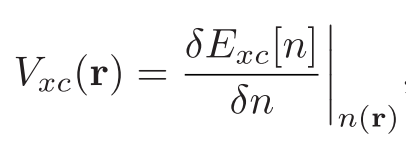As we described in the previous part it is the exchange and correlation potential. So, there is a functional, E_xc[n] that leads to the total energy. This functional is unknown but is related to the exchange and correlation potential.

How do we approximate this functional?

one example for the approximation:

5.1.The Local Density Approximation (LDA)

In this method, we consider the electrons as particles in a homogenous gas. A gas in a box with nuclei in it. We can calculate the exchange and correlation energy as a function of electron density, and also consider the repulsion btw particles in a homogenous gas model.
In fact, we consider the real material as a summation of local areas with infinitesimal width that has homogenous gas behavior.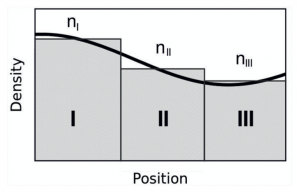(from ref. )(E_xc-HEG is well defined and calculated in )

By having the exchange and correlation energies, we can calculate the exchange and correlation potential (by derivation over density) and find the Kohn_Sham equation.

6. Self-consistent calculations (SCF)

Calculating the Kohn-Sham equation is not as straightforward as it is expected. Look at these sections: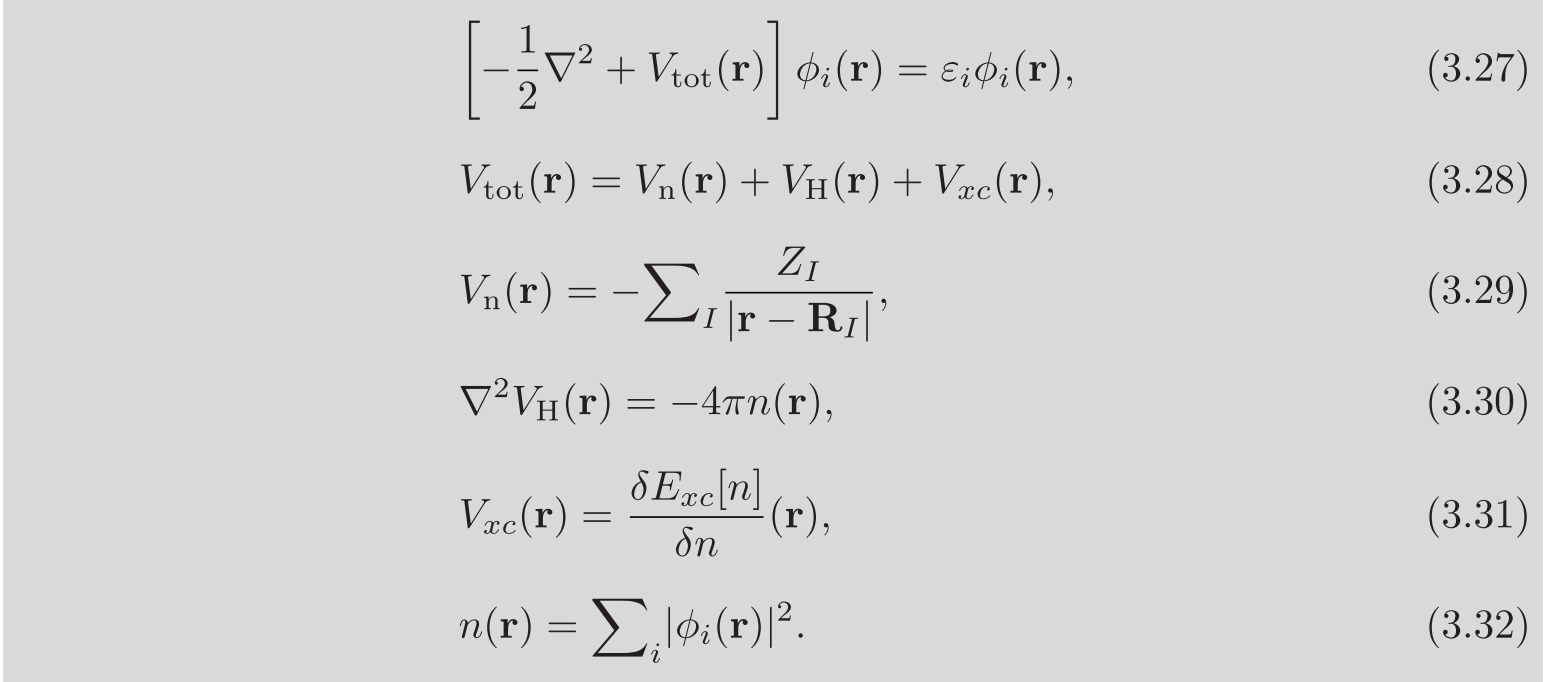• To find the ground state energy we need the electron density
• to find the electron density we need to solve the equation and find the wave function
• to find the wave function we need to have the total potentials
• and for finding the potentials we need to have the electron density

So, as we see we are trapped in a loop that to find one thing wee need another thing. But:

• First, we put the positions of the atom and GUESS the electron density as the atoms are not interacting with each other
• Then by this electron density find the potentials
• After that find the wave function
• And by this wave function calculate the new electron density
• If, the new electron density is as the same as the previous electron density, we keep it and calculate the energy
• If not, use this electron density and go to the 2nd step